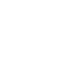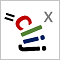# CLIJ2GPU accelerated image processing for everyone

CLIJ2 home

## generateDistanceMatrixAlongAxisComputes the distance in X, Y or Z (specified with parameter axis) between all point coordinates given in two point lists.

Takes two images containing pointlists (dimensionality n * d, n: number of points and d: dimensionality) and builds up a matrix containing the distances between these points.

Convention: Given two point lists with dimensionality n * d and m * d, the distance matrix will be of size(n + 1) * (m + 1). The first row and column contain zeros. They represent the distance of the objects to a theoretical background object. In that way, distance matrices are of the same size as touch matrices (see generateTouchMatrix). Thus, one can threshold a distance matrix to generate a touch matrix out of it for drawing meshes.

Categories: Graphs, Measurements

Availability: Available in Fiji by activating the update sites clij and clij2. This function is part of clijx_-0.32.0.1.jar.

### Usage in ImageJ macro

``````Ext.CLIJx_generateDistanceMatrixAlongAxis(Image coordinate_list1, Image coordinate_list2, Image distance_matrix_destination);
``````

Imprint